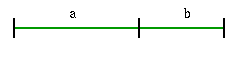#### You may also like### Gold Again

Without using a calculator, computer or tables find the exact values of cos36cos72 and also cos36 - cos72.### Pythagorean Golden Means

Show that the arithmetic mean, geometric mean and harmonic mean of a and b can be the lengths of the sides of a right-angles triangle if and only if a = bx^3, where x is the Golden Ratio.### Golden Triangle

Three triangles ABC, CBD and ABD (where D is a point on AC) are all isosceles. Find all the angles. Prove that the ratio of AB to BC is equal to the golden ratio.

# Golden Ratio

##### Age 16 to 18Challenge Level

The 'divina proporzione' or golden ratio, represented by the Greek letter phi, is derived from the equation below where $a$ and $b$ are parts of a line.$a+b:a=a:b$ i.e. $\frac{a+b}{a}=\frac{a}{b}=\Phi\ \quad$(phi)

If $b = 1$ show that $\Phi = a = (\sqrt 5 + 1 )/2 = 1.618034...$.

In the following equation what does $x$ equal?